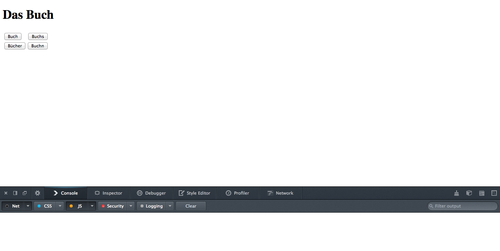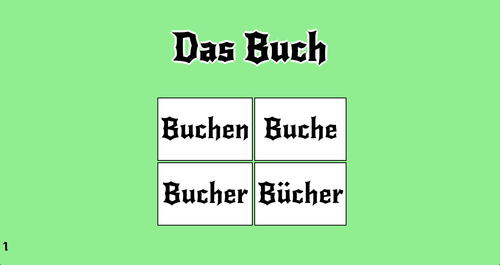Yesterday I talked about a simple Web App to test one’s ability on forming the plural of German Nouns. Recall, that I foresaw two possible implementations:

1. The application displays a word, say Buch, the the user needs to type in the correct answer, in this case Bücher.
2. The application displays a word, again Buch, and the user has the option of choosing the correct answer from, say, four answers, three of which are incorrect. So, Buch, Buchs, Buchen, and Bücher.

and implemented the first of these. Today, lets look at the second option. In Deutsch there are five main ways to form the plural of a noun:

1. Add -e, potentially an umlaut on the stem’s stressed vowel, i.e. der Schuh/die Schuhe, die Stadt/die Städte.
2. Add -er, potentially an umlaut on the stem’s stressed vowel, i.e. der Mann/die Männer
3. Add -n/-en (without any umlauts), i.e. die Blume/die Blumen.
4. Add -s, i.e. das Auto/die Autos.
5. No ending change, but potentially an umlaut, die Mutter/die Mütter.

The basic logic follows from yesterday’s game

``````//array of words (in their singular form)
var singularWords = ["Das Buch", "Der Mann", "Die Frau", "Das Kind"];
//and an array of their plural forms
var pluralWords = ["Bücher", "Männer", "Frauen", "Kinder"];

//variables to the question word, buttons, and score HTML elements
var buttons = [document.getElementById("button1"),
document.getElementById("button2"),
document.getElementById("button3"),
document.getElementById("button4")];
var scoreField = document.getElementById("score");
//scoreField.innerHTML = "0";

//a variable to note which word we are quizing
var questionIndex;
//and another to note the correct answer
//and a variable to keep count of the user''s score
var userScore = 0;

//initialize the game by setting up the next question
setUpNextQuestion();

//this function sets up the next question
function setUpNextQuestion()
{
//generate a random index from 0 to 3
questionIndex = Math.floor( Math.random()*4 );

//set the word at this position as the question word
questionWord.innerHTML = singularWords[questionIndex];

//the question word is of the form ''Das Kind'', we just want
''Kind'' for calculating random plurals
var questionWithoutArticle = singularWords[questionIndex].split(" ");

//generate a random answer from 0 to 3

//create an array of possible answers (initially unique)
var possibleAnswers = ["a", "b", "c", "d"];

//for the other elements, we generate random plurals
for(var k=0; k < 4; k++)
{
{
{
}
}
}

//finally update the buttons
for(var k=0; k < buttons.length; k++)
{
}
}

//this fuction checks the submitted answer
{
{
console.log("correct!");
userScore++;
}
else
{
console.log("incorrect");
}

//
scoreField.innerHTML = "" + userScore;

//setup next question
setUpNextQuestion();
}
``````

where hasDuplicates is a prototype for the Array class to check whether an Array contains duplicates or not

``````//a prototype for the Array class
//this method determines if the array contains duplicates
Array.prototype.hasDuplicates = function()
{
//console.log("Array.prototype.hasDuplicates");
for(var i = 0, n = this.length; i < n; i++)
{
for(var j = i+1; j < n; j++)
{
if( this[i] === this[j] )
{
return true;
}
}
}
return false;
}
``````

randomPluralEnding is function which takes a singular noun, and generates a random plural version, using the basic principles mentioned above

``````//this function returns a random plural for a given singular noun
function randomPluralEnding(singular)
{
//generate a random number and declare an ending variable
var randomEnding = Math.floor( Math.random()*5);
var ending = "";

switch( randomEnding )
{
case 0:
if( singular.slice(-1) === "e")
{
ending = "";
}
else
{
ending = "e";
}
break;
case 1:
if( singular.slice(-1) === "e")
{
ending = "r";
}
else
{
ending = "er";
}
break;
case 2: //singular ending in "n" is given "en" ending
if( singular.slice(-1) === "n")
{
ending = "en";
}
else //otherwise 50-50 "n" vs "en"
{
if( Math.random() > 0.5 )
{
ending = "n";
}
else
{
ending = "en";
}
}
break;
case 3:
ending = "s";
break;
case 4:
ending = "";
break;
}
return singular + ending;
}
``````

So far it doesn’t consider any umlauts, just some basic logic that ‘n’ cannot follow an ‘‘n’ (i.e. Mannn) or ‘‘er’/’‘e’ after an ‘‘e’ (i.e. Blumeer). The HTML element is simply a header and table of buttons. The header displays the question, and the button the potential answers. The user can either click the buttons or press the digits 1-4 to submit an answer.Clearly this is a little boring, but some CSS can spice it up!Here the answer buttons are in a table. I haven’t figured out yet how to have a fixed column size which is relative to the screen width and height.